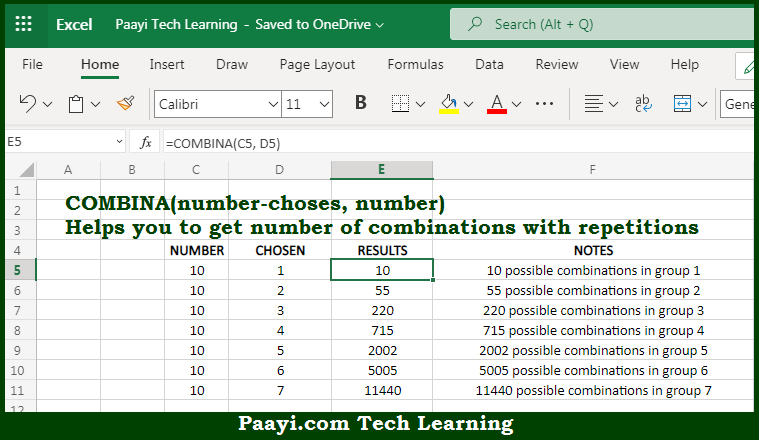# Learn How to Use Microsoft Excel COMBINA Function

Written by | 0 Comments | 576 Views

In this article, you will learn how to use the Microsoft Excel COMBINA function and its prime function in Microsoft Excel. You will also get to know the Microsoft Excel COMBINA function return value and syntax with the help of some examples.

Microsoft Excel COMBINA Function

The main function of the Microsoft Excel COMBINA function is to get the number of combinations with repetitions. So, with the help of COMBINA function, you can able to return the number of combinations where the repetitions are allowed. In case you want to count combinations that don't allow combinations you can use COMBIN function. So, with the help of the Microsoft Excel COMBINA function, you can easily return the number of combinations where the repetitions are allowed.

Return Value of COMBINA Function

The return value will be the number of combinations as an integer.

Syntax of COMBINA Function

=COMBINA(number, number-chosen)

Where the arguments:

• number: This is the total number of items.
• number-chosen: This is the number of items of each combination.

How to Use Microsoft Excel COMBINA Function?Microsoft Excel COMBINA function is used to return the number of combinations with repetitions for a given number of items. The combination can be the group of items, where order does not matter. There are two types of combinations operations as given below:

• Combinations that don't allow repetitions.
• Combinations that allow repetitions.

The COMBINA function allows repetitions. To count combinations that do not allow repetitions, use the COMBIN function. So, with the help of the Microsoft Excel COMBINA function you can easily round up the number to the nearest multiple.